### 机器学习09：逻辑回归详解

• 发布时间：2017年3月28日 09:56
• 作者：杨仕航

《机器学习实战》书中第5章讲的是逻辑回归（Logistic回归）。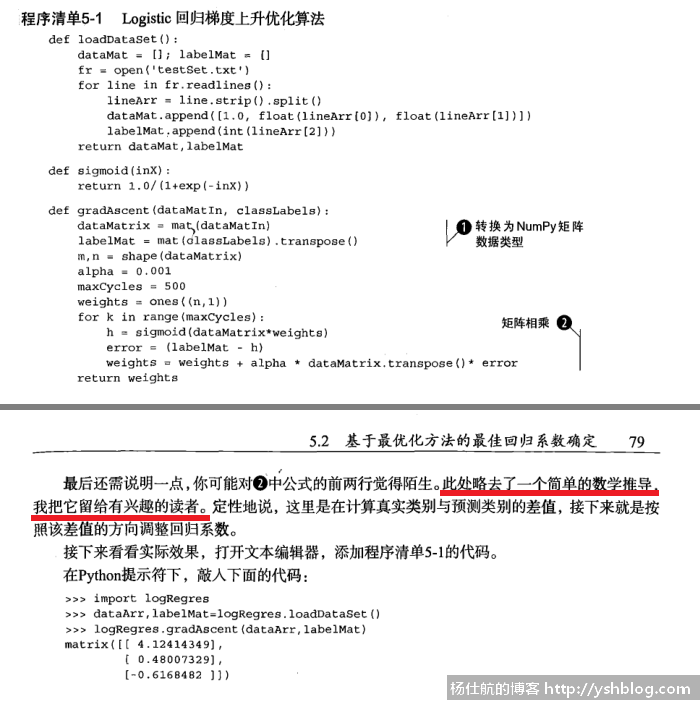#### 1、什么是逻辑回归（Logistic回归）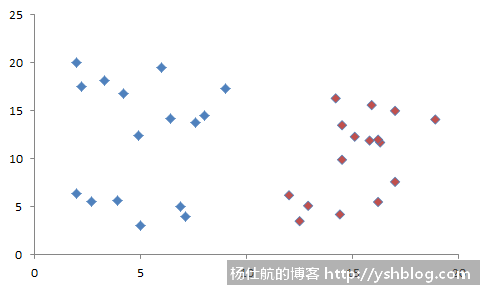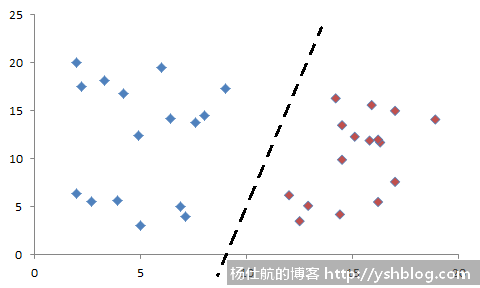z = θ0X01X12X2+...+θnXn , 其中X0=1#### 2、利用Sigmoid函数分类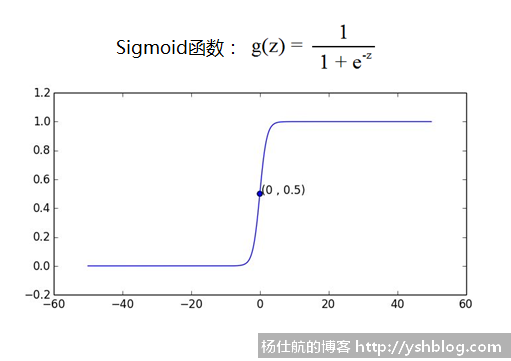#### 3、如何拟合线性方程（边界线）

1）概率是已知参数或条件，预测或观测可能发生的结果；

2）似然是已知某些观测到的结果，估计相关的参数或条件。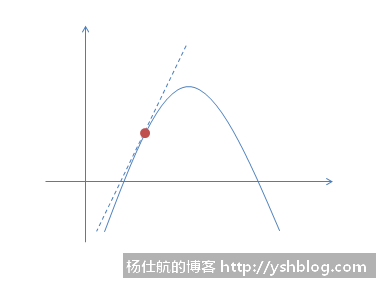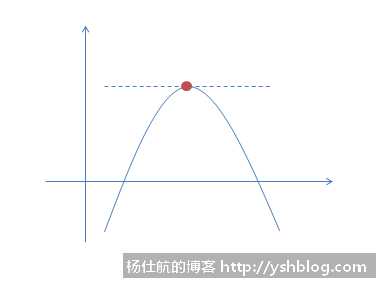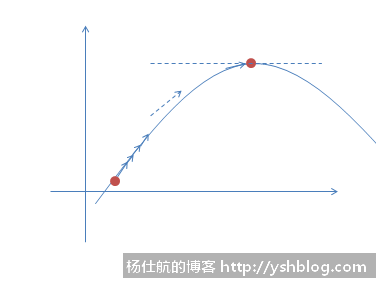θ:= θj + α*θj′

#### 4、逻辑回归的最大似然估计

P(y=1 | x;θ) = hθ(x)

P(y=0 | x;θ) = 1 - hθ(x)

P(y | x;θ) = hθ(x)y(1 - hθ(x))(1-y)L(θ)为θ的参数的分布函数。也是我们梯度递增所需要的导数的原函数。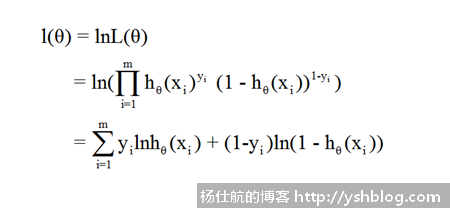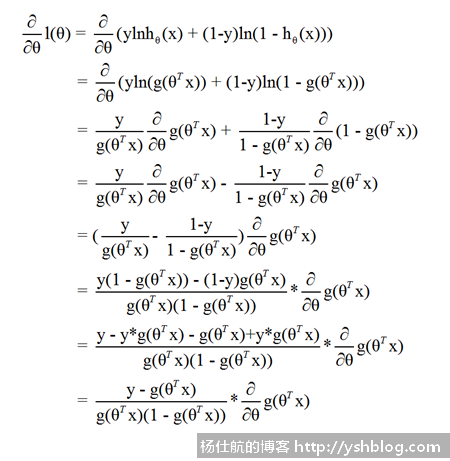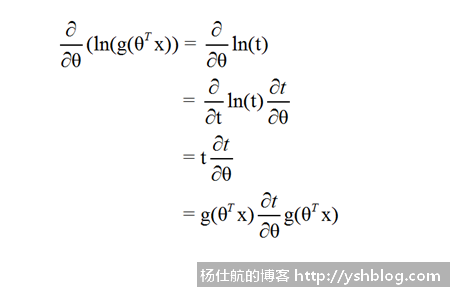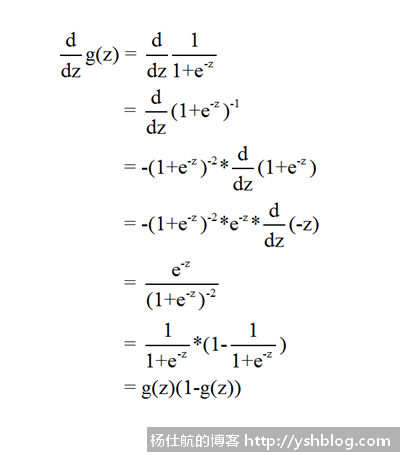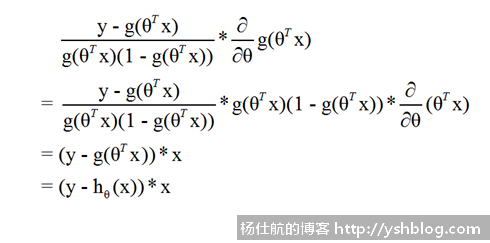#### 5、Python代码实现逻辑回归分类

```#coding:utf-8
import numpy as np

#获取训练集
def load_dataset(filename='testSet.txt'):
dataset = []
labels = []
with open(filename, 'r') as f:
line = f.readline()
while line != '':
data = line.strip().split()
dataset.append([1., float(data), float(data)])
labels.append(int(data))
line = f.readline()

return dataset, labels```

```#S型函数
def sigmoid(x):
return 1./(1+np.exp(-x))```

Logistic逻辑回归实现代码如下：

```#拟合参数
def grad_ascent(dataset, labels, alpha=0.001, max_cycles=500):
dataset = np.mat(dataset)
labels = np.mat(labels).transpose()

m, n = np.shape(dataset)
weights = np.ones((n, 1)) #初始化参数
trans_dataset = dataset.transpose()

for k in range(max_cycles):
#使用S型函数分类
h = sigmoid(dataset*weights)

#梯度上升
weights = weights + alpha*trans_dataset*(labels-h)
return weights```

```if __name__ == '__main__':
dataset, labels = load_dataset()
weights = grad_ascent(dataset, labels)
print(weights)```

```#coding:utf-8
import matplotlib.pyplot as plt

#画图
def plot_graph(dataset, labels, weights):
dataset = np.array(dataset)
m, n = np.shape(dataset)

#数据分组
r_x1 = []
r_x2 = []
g_x1 = []
g_x2 = []

for i in range(m):
if labels[i] == 1:
r_x1.append(dataset[i, 1])
r_x2.append(dataset[i, 2])
else:
g_x1.append(dataset[i, 1])
g_x2.append(dataset[i, 2])

#画数据点
fig = plt.figure()
ax = fig.add_subplot(111)
ax.scatter(r_x1, r_x2, s=30, c='red', marker='s')
ax.scatter(g_x1, g_x2, s=30, c='green')

#画边界线
x1 = np.arange(-3., 3., 0.1)
x2 = (-weights - weights*x1)/weights
ax.plot(x1, x2.transpose())

plt.xlabel('x1')
plt.ylabel('x2')
plt.show()```

0 = θ01X12X2

X= (- θ1X0)/θ2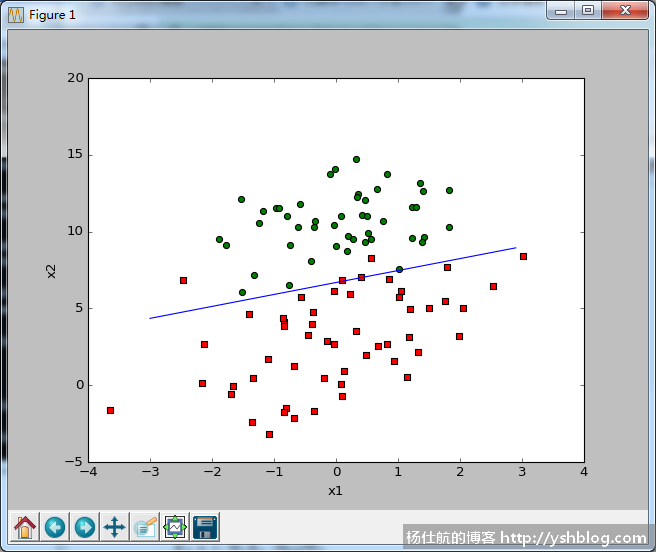#### 评论列表2017-03-28 10:03